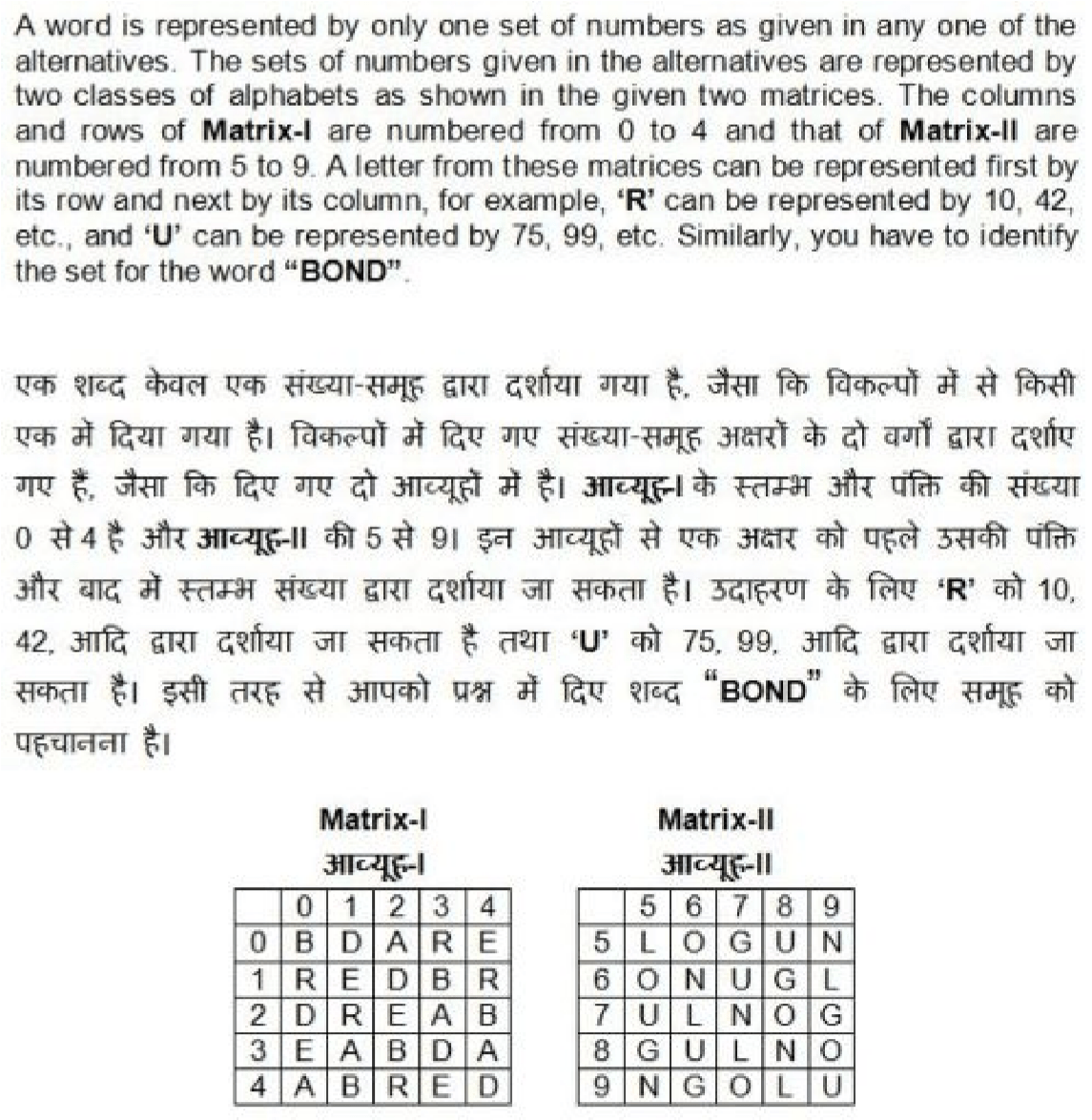# SSC Online Exam free Practice: General Intelligence and Reasoning: CPO, CGL levelStaff Selection Commission (SSC) General Intelligence and Reasoning CPO level Free Online Mock Exam/ Test practice

Number pf Questions : 50 Time Duration : 30 Minutes

6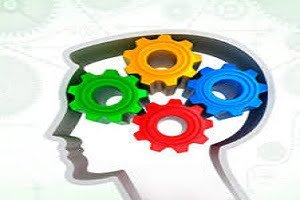SSC General Intelligence and Reasoning CPO CGL

1 / 50

In the following question, select the related word from the given alternatives.

Farmer : Field : : Painter : ?

2 / 50

In the following question, select the related word from the given alternatives.

Sunday : Thursday : : Wednesday : ?

3 / 50

In the following question, select the related letters from the given alternatives.

MN : OL : : SH : ?

4 / 50

In the following question, select the related letters from the given alternatives.

STOP : TRVT : : ? : ?

5 / 50

- In the following question, select the related number from the given alternatives.

48 : 216 : : 64 : ?

6 / 50

In the following question, select the related number from the given alternatives.

36 : 27 : : 196 : ?

7 / 50

In the following question, select the odd word pair from the given alternatives.

8 / 50

In the following question, select the odd word pair from the given alternatives.

9 / 50

In the following question, select the odd letters from the given alternatives.

10 / 50

In the following question, select the odd letters from the given alternatives.

11 / 50

In the following question, select the odd number from the given alternatives.

12 / 50

In the following question, select the odd number pair from the given alternatives.

13 / 50

Arrange the given words in the sequence in which they occur in the dictionary.

1. Reputation
2. Reptile
3. Republic
4. Replicate

14 / 50

Arrange the given words in the sequence in which they occur in the dictionary.

1. Habit
2. Habitat
3. Handle
4. Hammer

15 / 50

A series is given with one term missing. Select the correct alternative from the given ones that will complete the series.

B, G, N, W, ?

16 / 50

A series is given with one term missing. Select the correct alternative from the given ones that will complete the series.

B, E, I, S, K, ?

17 / 50

In the following question, select the missing number from the given series.

67, 70, 74, 77, 81, 84, ?

18 / 50

In the following question, select the missing number from the given series.

6, 19, 54, 167, 494, ?

19 / 50

In a row of cars, red car is 14th from left and 23rd from right. How many cars are there in the row?

20 / 50

In a row of people, there are 12 people before Q. There are 4 people between P and Q. There are 15 people between Q and S. If there are 8 people between S and R, then how many minimum people are there in the row?

21 / 50

If 'P 3 Q' means 'Q is daughter of P', 'P 5 Q' means 'Q is son of P', 'P 7 Q' means 'P is sister Q', 'P 9 Q' means 'P is brother of Q'. Which of the following expression indicates A is nephew of D?

22 / 50

In the following question, select the word which cannot be formed using the letters of the given word.

ENCOURAGING

23 / 50

In the following question, select the word which cannot be formed using the letters of the given word.

LANGUAGE

24 / 50

In a certain code language, "RESTED" is written as "SDTSFC" and "POINTS" is written as "QNJMUR". How is "BANNED" written in that code language?

25 / 50

In a certain code language, "CONGO" is written as "RZPRD" and "TREAT" is written as "UQGWX". How is "PHONE" written in that code language?

26 / 50

If “@” denotes “added to”, “#” denotes “multiplied by”, “®” denotes “divided by” and “%” denotes “subtracted from”, then which of the following equation is true?

27 / 50

If "A" denotes "added to", "B" denotes "subtracted from", "C" denotes "multiplied by" and "D" denotes "divided by", then which of the following equation is true?

28 / 50

If 6 # 8 = 10 and 5 # 12 = 13, then 9 # 40 = ?

29 / 50

If 7 (110) 4 and 19 (930) 12, then what is the value of 'A' in 16 (A) 9?

30 / 50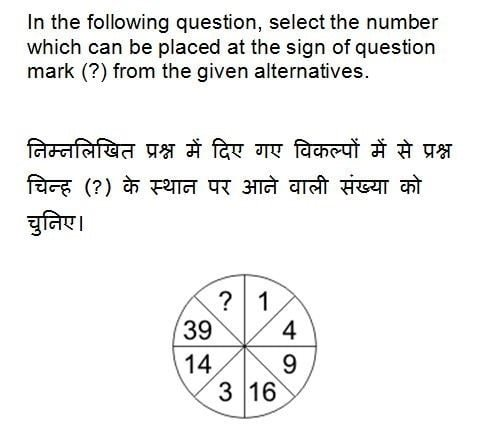31 / 50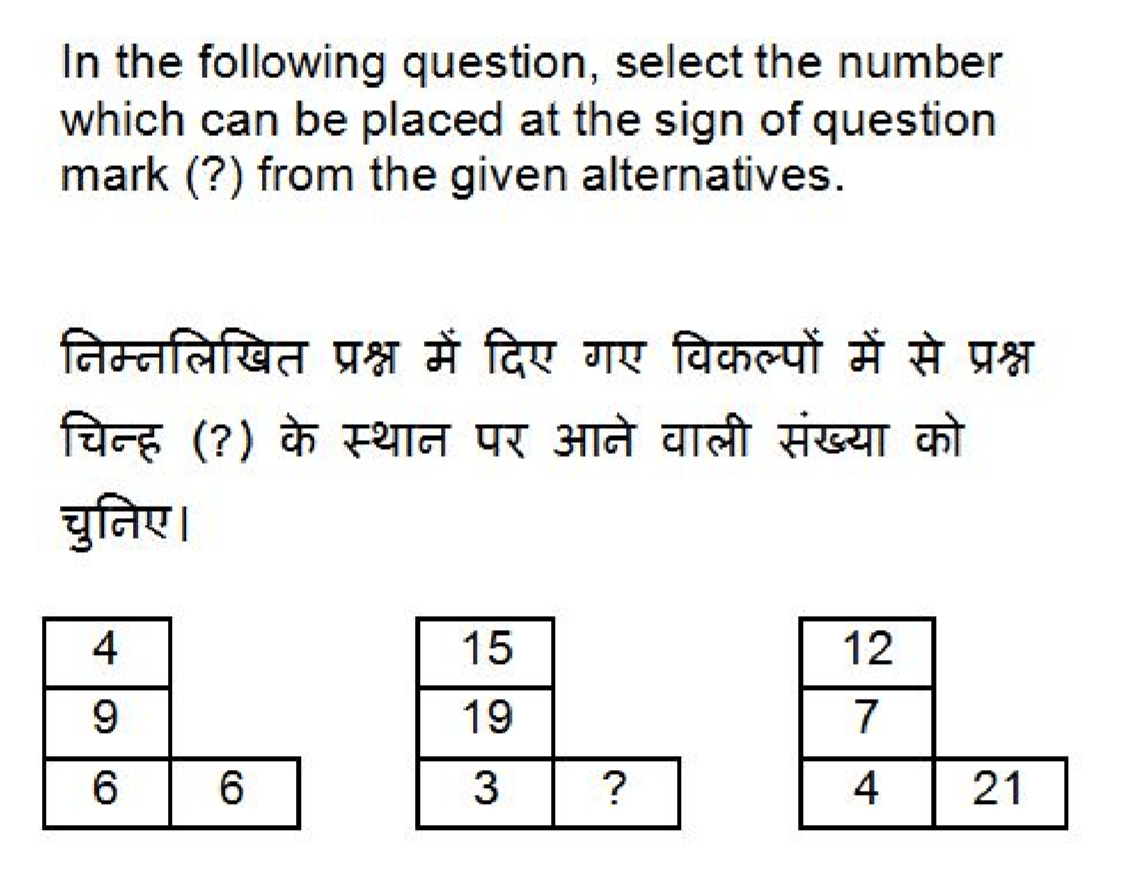32 / 50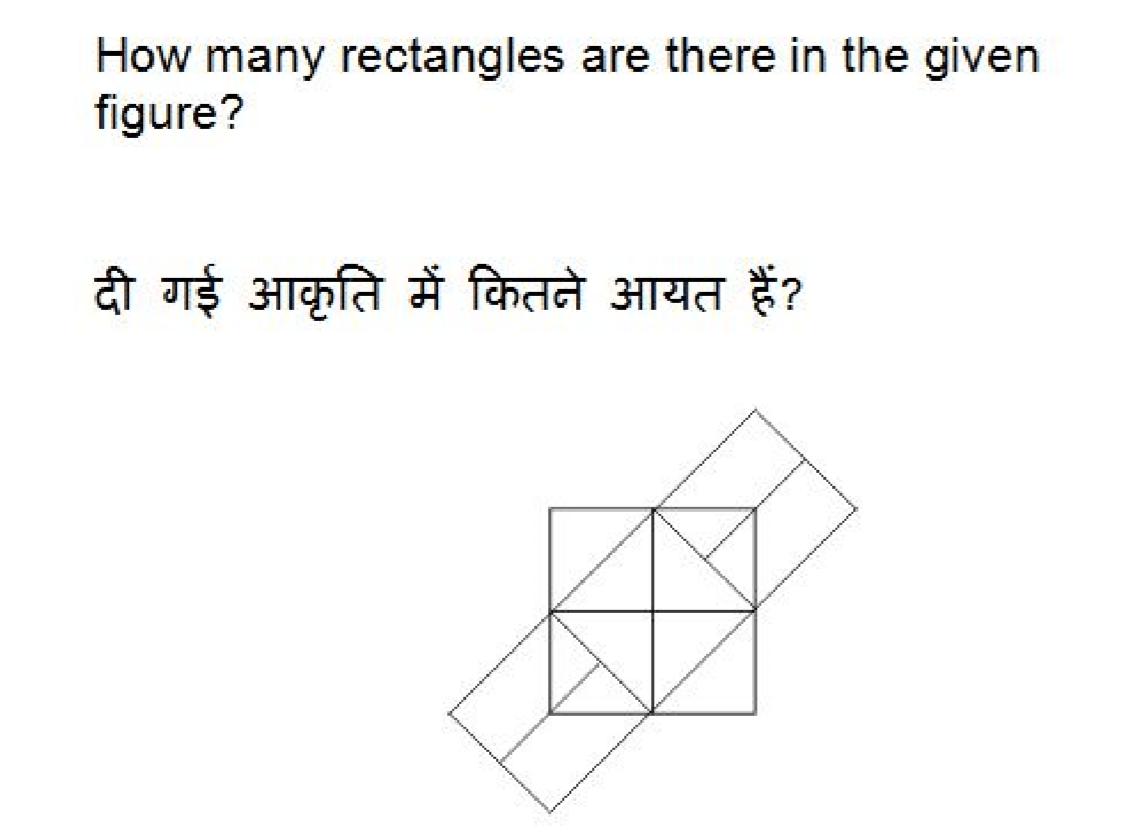33 / 50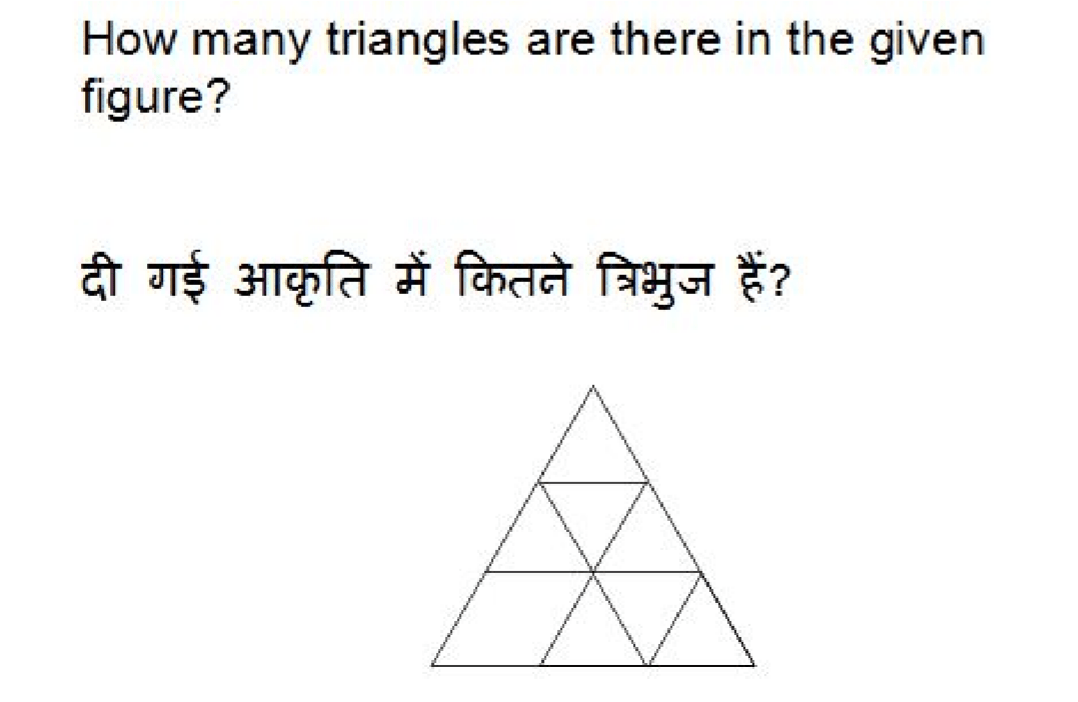34 / 50

In each of the following question below are given some statements followed by some conclusions. Taking the given statements to be true even if they seem to be at variance from commonly known facts, read all the conclusions and then decide which of the given conclusion logically follows the given statements.

Statements:

1. Some clothes are white II. Some white are flags. III. No flag is straight.

Conclusions:

1. No cloth is straight.
2. Some white are straight

Some flags are clothes

35 / 50

In each of the following question below are given some statements followed by some conclusions. Taking the given statements to be true even if they seem to be at variance from commonly known facts, read all the conclusions and then decide which of the given conclusion logically follows the given statements.

Statements:

1. All pages are yellow.
2. All yellow are newspaper.
• Some newspapers are national.

Conclusions:

I.  Some national are yellow.

II.  Some newspapers are pages.

III. No page is national.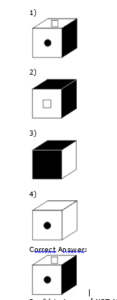36 / 50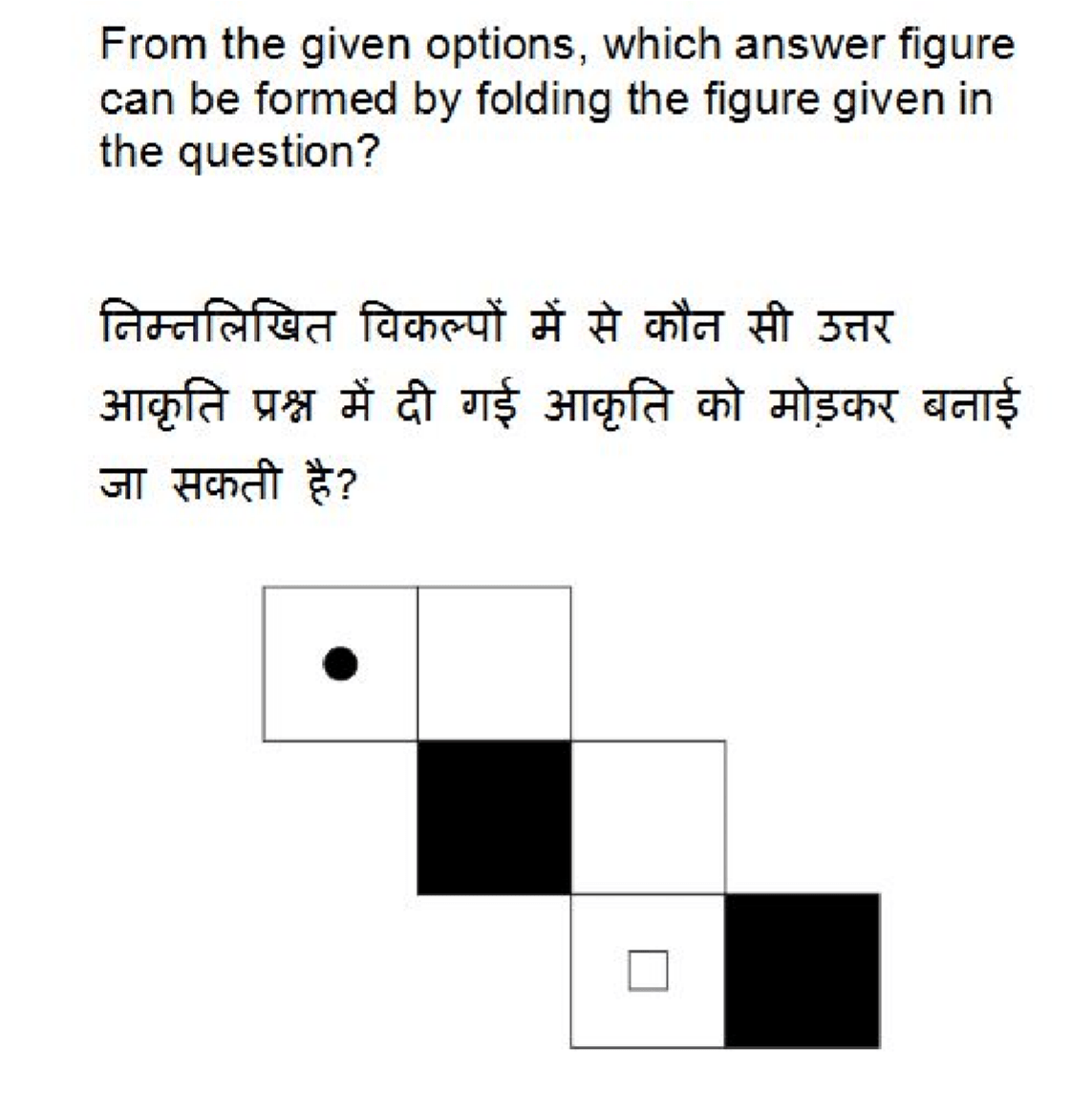37 / 50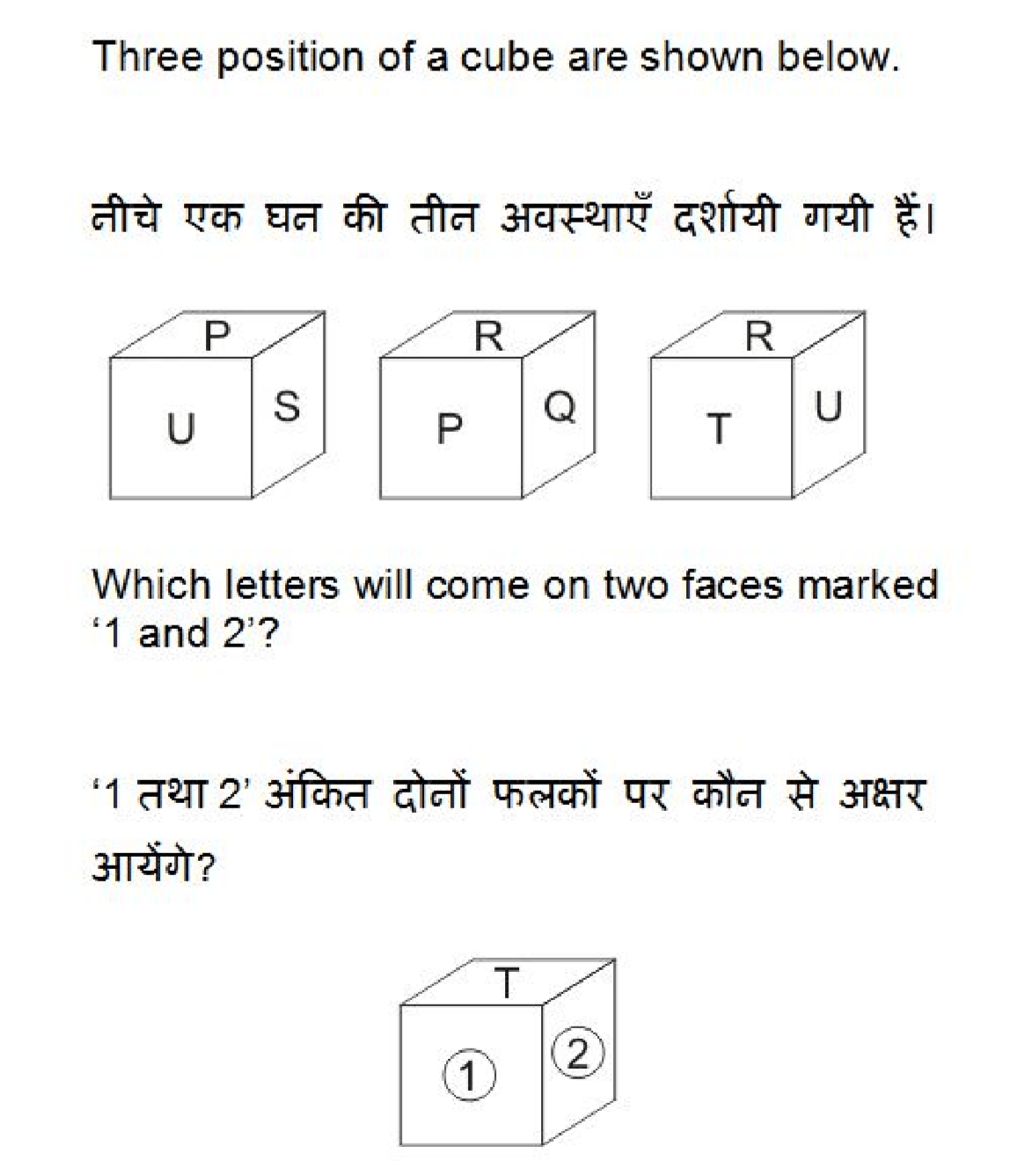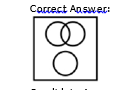38 / 50

Identify the diagram that best represents the relationship among the given classes. Yellow, Vegetables, Red Blood

check Hint in left corner i for Answer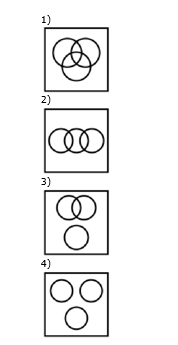39 / 50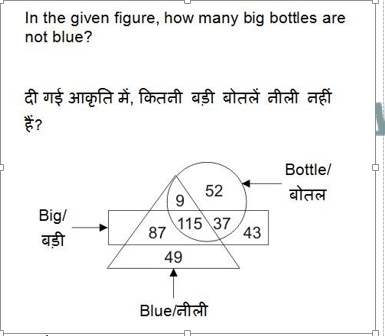40 / 50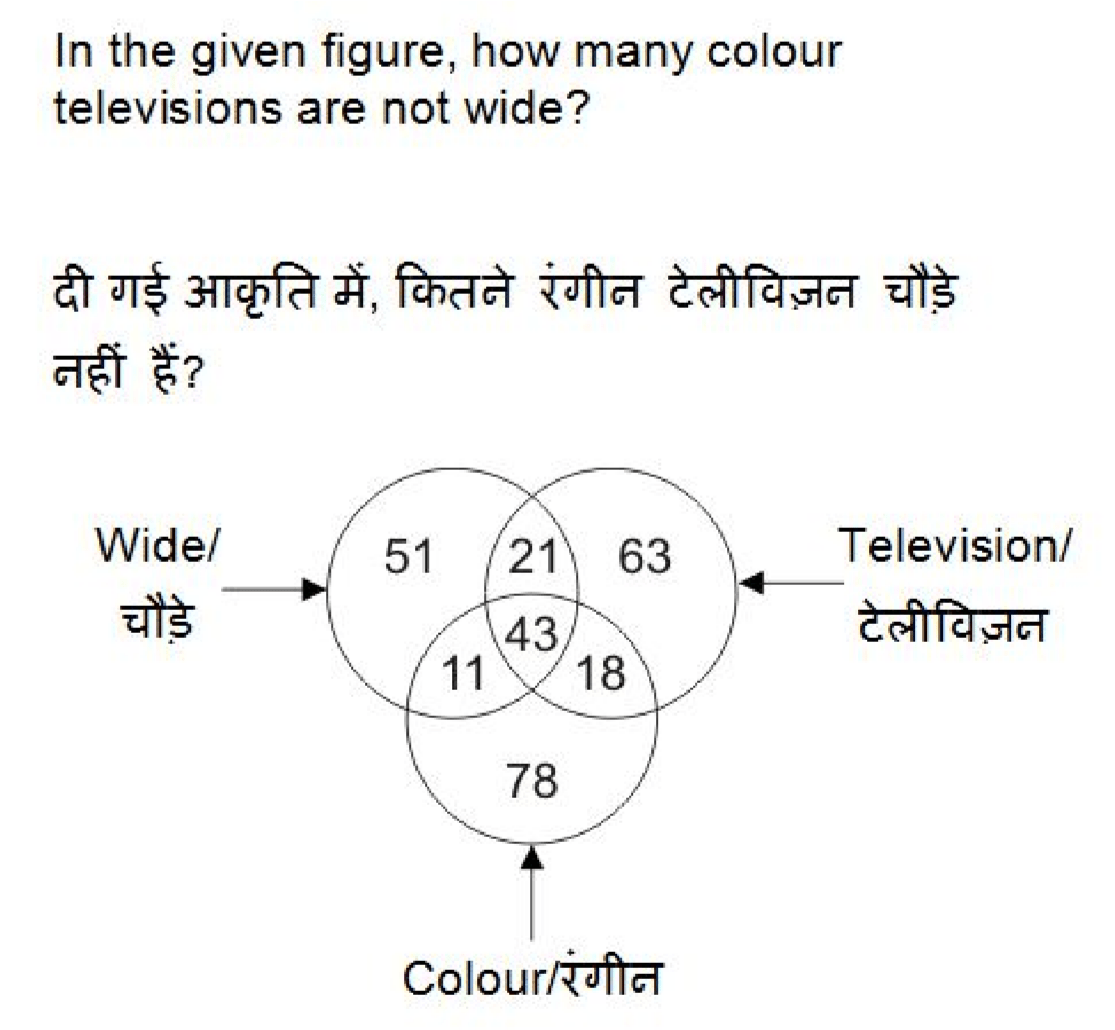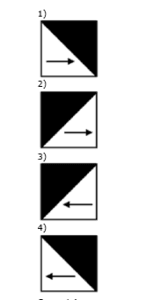41 / 50

Check Hint in Upper Left corner !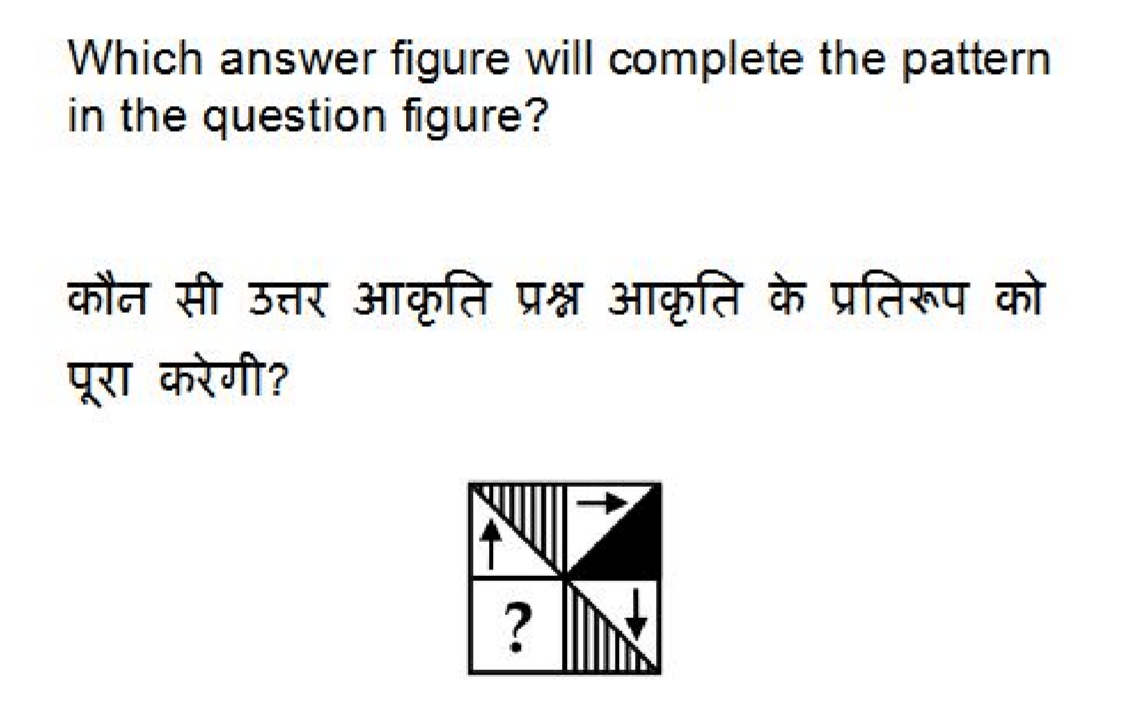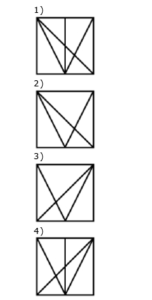42 / 50

Check Hint for images  in Upper left corner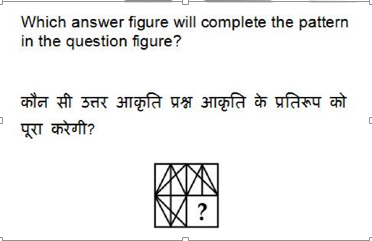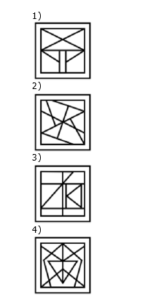43 / 50

Check left corner hint for option images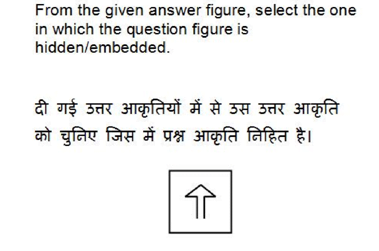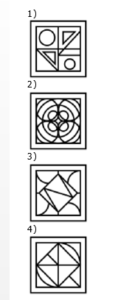44 / 50

Check Hint ; for option images in upper left corner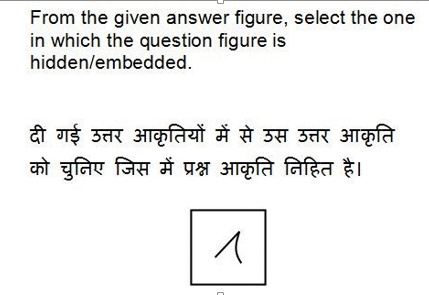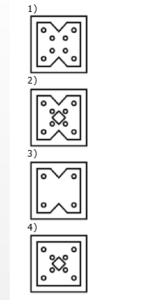45 / 50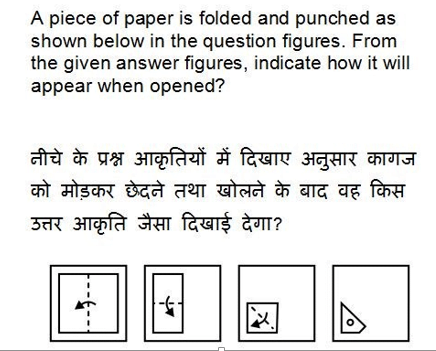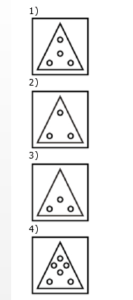46 / 50

Check hint button for option image in left corner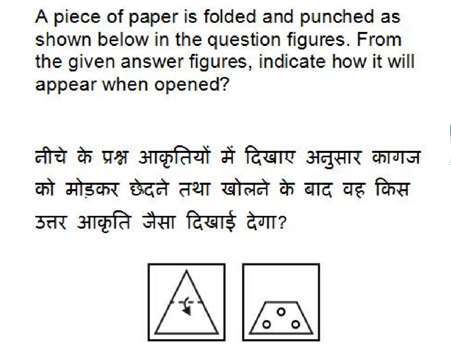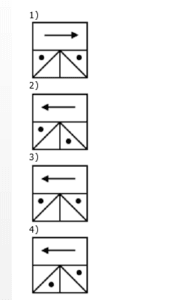47 / 50

click hint button for option images in left corner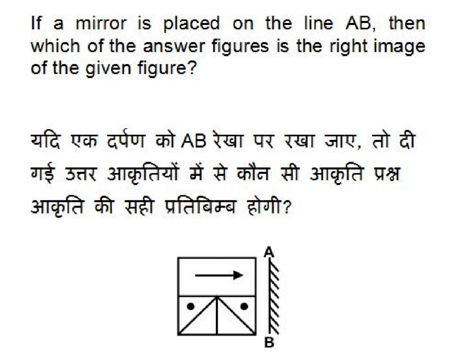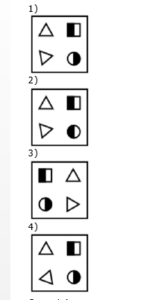48 / 50

Click Hint Icon for option images : Left corner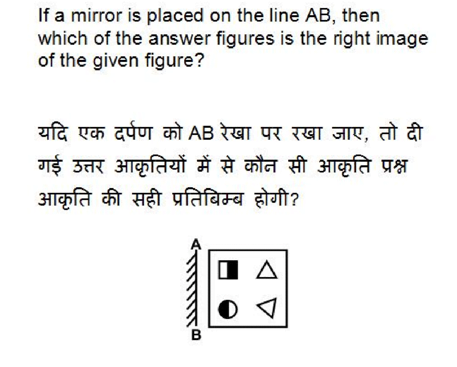49 / 50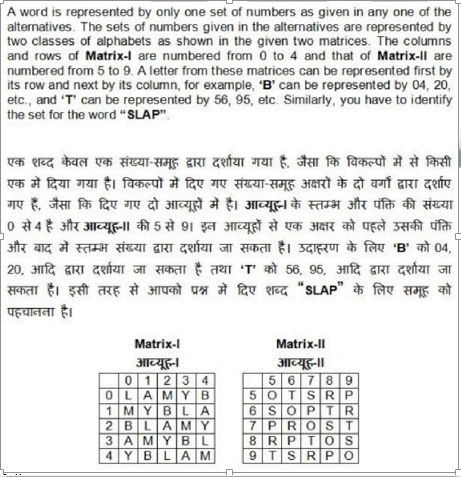50 / 50